#### You may also like### Ball Bearings

If a is the radius of the axle, b the radius of each ball-bearing, and c the radius of the hub, why does the number of ball bearings n determine the ratio c/a? Find a formula for c/a in terms of n.### Air Routes

Find the distance of the shortest air route at an altitude of 6000 metres between London and Cape Town given the latitudes and longitudes. A simple application of scalar products of vectors.### Over-booking

The probability that a passenger books a flight and does not turn up is 0.05. For an aeroplane with 400 seats how many tickets can be sold so that only 1% of flights are over-booked?

# A Very Shiny Nose?

##### Age 16 to 18Challenge Level

Well done to Anya from Tanglin Trust School in Singapore and Emily from Bishop's Wordsworth's Grammar School in the UK who found a complete solution to this problem. We also received some partial or incorrect solutions which contained interesting maths, so these are also shown below.

Anya from Tanglin Trust School in Singapore used the floor function to write the number of bacteria as a function of time ($x$) (click to enlarge):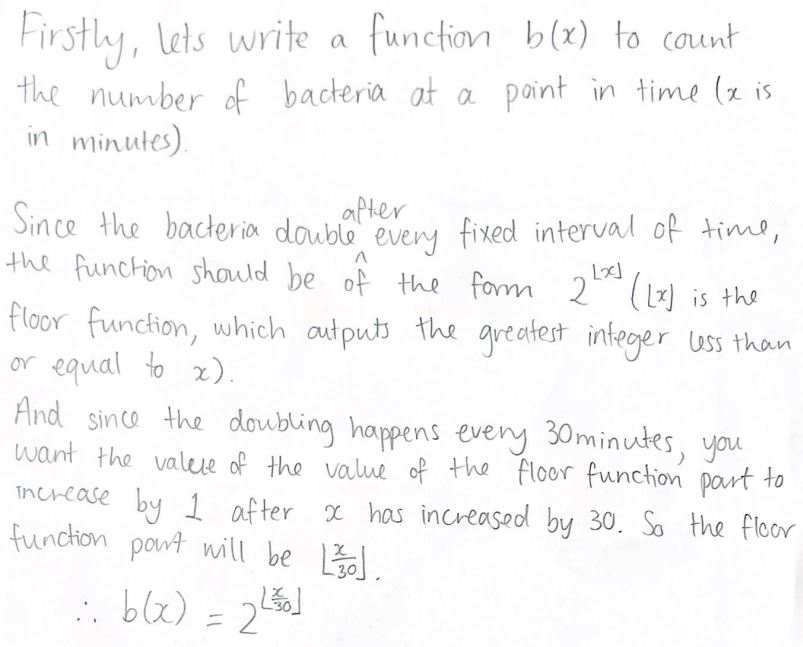Anya then used this to estimate the number of bacteria: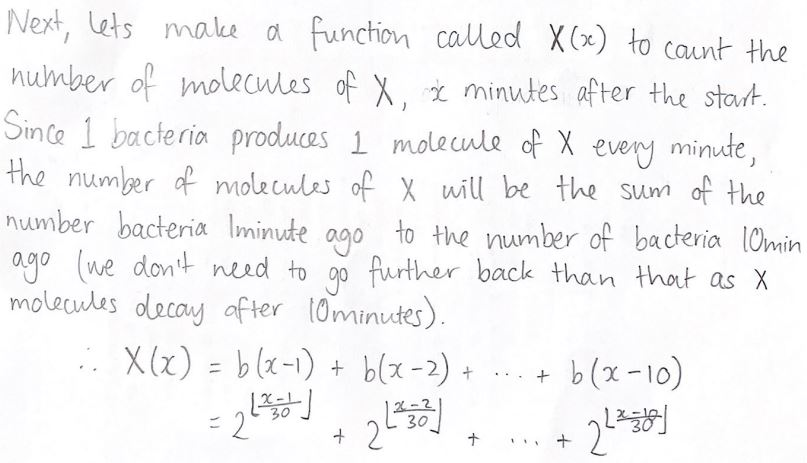In fact, only half of substance X decays after 10 minutes. Anya assumes that all of it disappears after 10 minutes. This means Anya's total number of molecules of X will be an underestimate.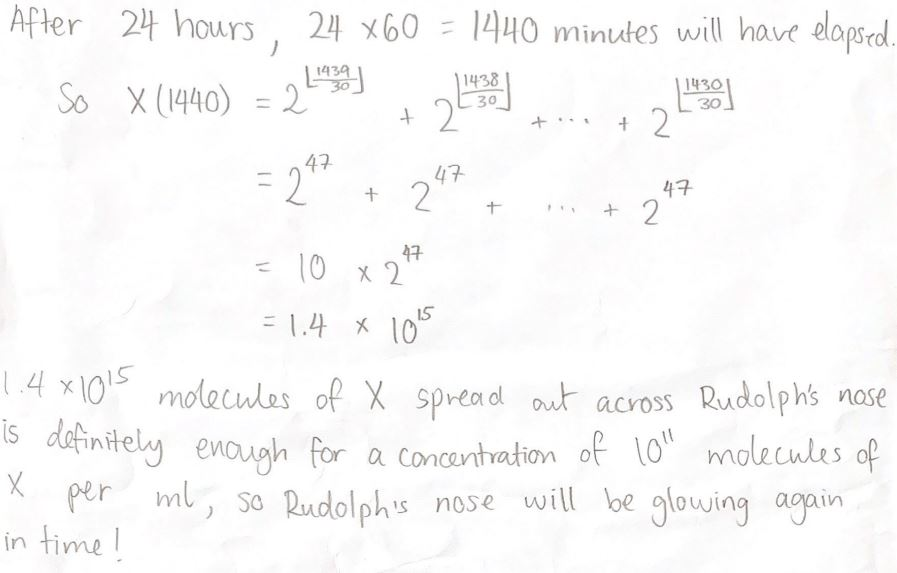Anya has assumed that the volume of Rudolph's nose which is holds the bacteria and molecules of X is at least $1.4\times10^{15}\div10^{11}=14,000$ millilitres. That's probably reasonable!

Emily from  Bishop's Wordsworth's Grammar School in the UK used an iterative formula to estimate the number of bacteria every half hour (click to enlarge):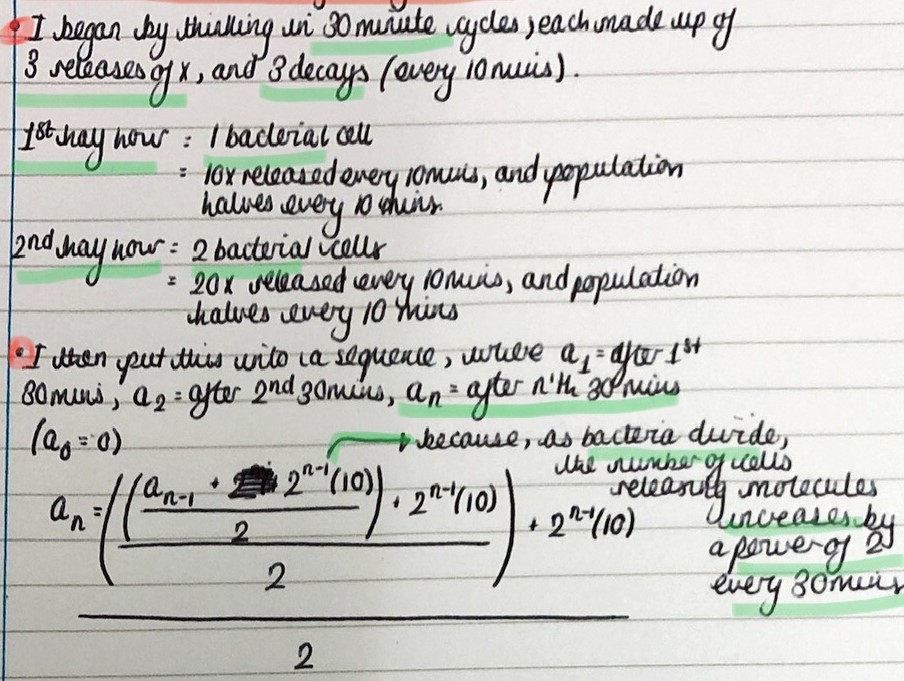Emily's formula includes adding $2^n\times10$ for the molecules released in the first $10$ minutes (one molecule per bacterium per minute), and then dividing by $2$ after $10$ minutes have passed. Then in the next $10$ minutes, Emily adds $2^n\times10$ again and then divides by $2$ again. Emily repeats this for the third set of $10$ minutes in the half hour. This is an estimate, since in fact the molecules released one minute apart decay on a slightly different half-life cycle, but it is a good estimate.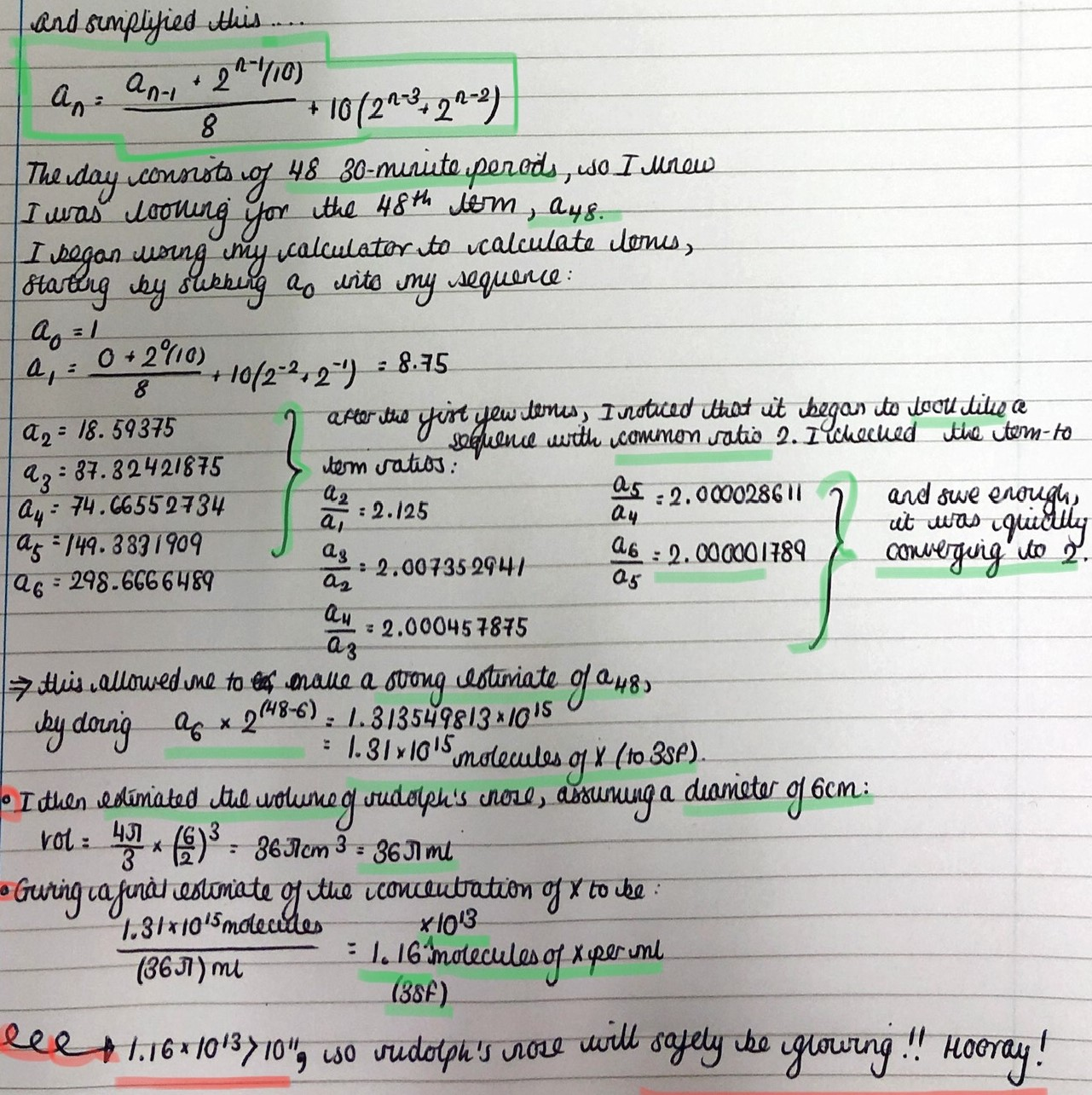Isabella from King's College London Maths School tried to represent the situation as a system of differential equations: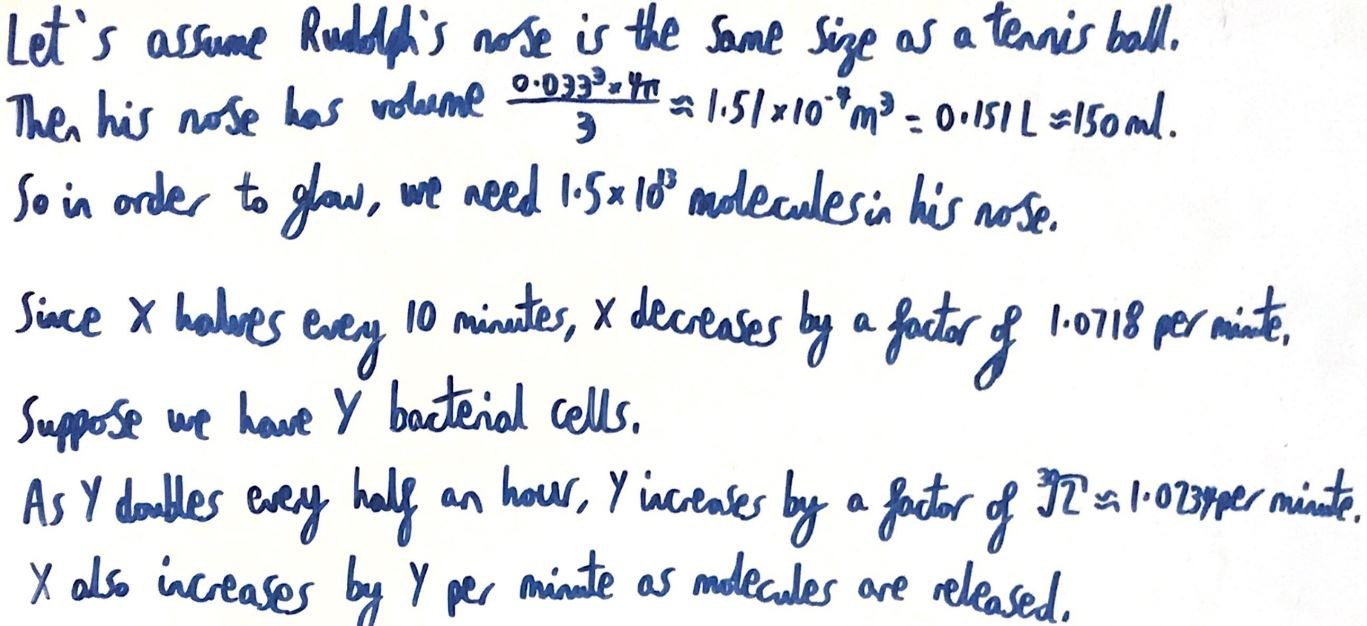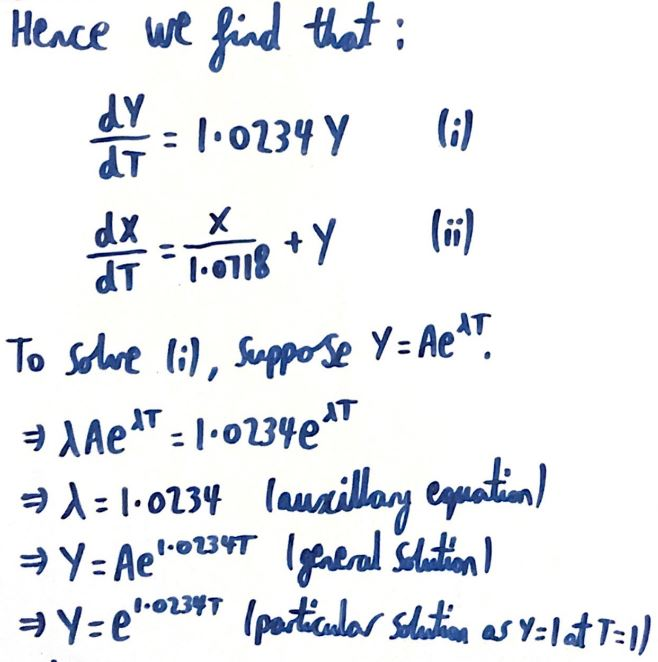This is beautiful and a great way of expressing the situation. However, derivatives, or gradient, refer to the additive change in $Y$ every time $T$ increases, not the multiplicative change or "scale factor". This means you can't say that $$\frac{\text{d}Y}{\text{d}T}=1.0234Y$$ However, you can say that the rate of change of $Y$ is proportional to $Y$, so $$\frac{\text{d}Y}{\text{d}T}=kY$$ You can then use Isaella's method of supposing $Y=Ae^{\lambda}T.$ Differentiate this expression for $Y$ with respect to $T$ to see how $k,A$ and $\lambda$ are related, and then use $Y=1$ when $T=0$ and $Y=2$ when $T=30$ to find $A$ and $\lambda$.

In the equation for $\dfrac{\text{d}X}{\text{d}T},$ you can do the same for the first term. However, you cannot solve this equation easily: it is not separable. Isabella went on to "integrate" one term at a time, but the beauty of the system is that the molecules begin to decay as soon as they are emitted. Treating the terms separately does not capture this - the first term represents decay of $X$ and the second term represents creation of $X$ and if they are treated separately, the newly created molecules do not decay.